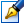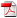##Symbols of some algebras of singular integral operators and the problems of regularization

 Author: Galina Vornicescu Degree: doctor of physics and mathematics Speciality: 01.01.01 - Mathematical analysis Year: 2004 Scientific adviser: Vasile Neagudoctor habilitat, professor, Moldova State University Institution: Scientific council:

### Status

The thesis was presented on the 29 November, 2004
Approved by NCAA on the 23 December, 2004

### Abstract0.19 Mb / in romanian

### Summary

The thesis is devoted to the following problems of the theory of integral singular operators (i.s.o.): construction of i.s.o. with shift and complex conjugation in the case of piecewise continuous coefficients; establishing of Lp Noetherian conditions and formulas for calculation of the index of i.s.o. with Carleman shift and complex conjugation; regularization of i.s.o. with shift and complex conjugation.

In solving of these problems the Banach algebras (noncommutative) generated by i.s.o. with shift and complex conjugation were studied and the notion of equivalence of two Banach algebras is introduced. It is proved that the algebra of operators with shift or with complex conjugation is equivalent to the algebra of i.s.o. does not containing the shift operators and complex conjugation. The form of a symbol was obtained in dependence of the space; of the coefficients of operators and of the contour of integration. It is shown that the symbol represents a functional matrix of the second order defined on R × Γ and is not depending from its representation in the form of sum of products of operators from a given algebra. Also, it is shown how are defined the symbols of operators of a closure algebra and the problem of symbol extension is solved. As a result of investigation of these algebras the Noether’s theorems were proved for i.s.o. with shift and complex conjugation and was obtained the formula for calculation of the index of the Noether operators.

The Noether conditions were established by regularization of i.s.o. with shift and the conditions by which i.s.o. with shift or with complex conjugation admits an equivalent regularization are found. The problem of spectrum and of solving some singular integral equations by method of direct factorization and by method of reduction of this equation to a system of singular equations which can be solved by method of factorization is considered. The results obtained in the thesis can be applied for further investigation of integral equations generated by i.s.o. with shift and complex conjugation. The method of construction of the matrix symbol of noncommutative algebras of i.s.o. developed in the thesis can be applied by investigation of more general operators: i.s.o. containing a finite number of involute operators, as well by studying of generalized Riemann problems for analytical functions.

The thesis is written in Romanian.